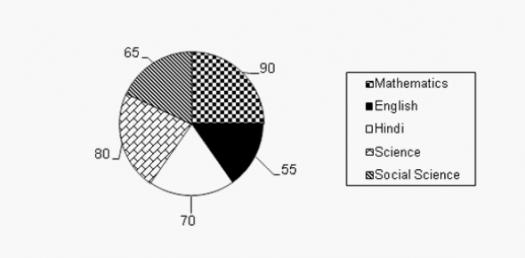# Pie And Line Chart Test! Trivia Questions Quiz

10 Questions | Attempts: 1736
ShareSettings.

• 1.
The following pie-chart shows the percentage distribution of the expenditure incurred in publishing a book. Study the pie-chart and answer the questions based on it. - If for a certain quantity of books, the publisher has to pay Rs. 30,600 as printing cost, then what will be the amount of royalty to be paid for these books? -
• A.

Rs. 19,450

• B.

Rs. 21,200

• C.

Rs. 22,950

• D.

Rs. 26,150

• 2.
What is the central angle of the sector corresponding to the expenditure incurred on Royalty? -
• A.

15 Degrees

• B.

24 Degrees

• C.

54 Degrees

• D.

48 Degrees

• 3.
The price of the book is marked 20% above the C.P. If the marked price of the book is Rs. 180, then what is the cost of the paper used in a single copy of the book?
• A.

Rs. 36

• B.

Rs. 37.50

• C.

Rs. 42

• D.

Rs. 44.25

• 4.
If 5500 copies are published and the transportation cost on them amounts to Rs. 82500, then what should be the selling price of the book so that the publisher can earn a profit of 25%?
• A.

Rs. 187.50

• B.

Rs. 191.50

• C.

Rs. 175

• D.

Rs. 180

• 5.
Royalty on the book is less than the printing cost by:
• A.

5%

• B.

33 1/5%

• C.

20%

• D.

25%

• 6.
Study the following line graph and answer the questions. Exports from Three Companies Over the Years (in Pesetas) For which of the following pairs of years the total exports from the three Companies together are equal?
• A.

1995 and 1998

• B.

1996 and 1998

• C.

1997 and 1998

• D.

1995 and 1996

• 7.
Average annual exports during the given period for Company Y is approximately what percent of the average annual exports for Company Z?
• A.

87.12%

• B.

89.64%

• C.

91.21%

• D.

93.33%

• 8.
In which year was the difference between the exports from Companies X and Y the minimum?
• A.

1994

• B.

1995

• C.

1996

• D.

1997

• 9.
What was the difference between the average exports of the three Companies in 1993 and the average exports in 1998? -
• A.

15.33

• B.

18.67

• C.

20

• D.

22.17

• 10.
In how many of the given years, were the exports from Company Z more than the average annual exports over the given years?
• A.

2

• B.

3

• C.

4

• D.

5

## Related TopicsBack to top
×

Wait!
Here's an interesting quiz for you.